# Name ALL lines and segments Name all rays

• Slides: 23Name ALL lines and segments.• Name all rays. • Are ray LP and ray PL opposite rays?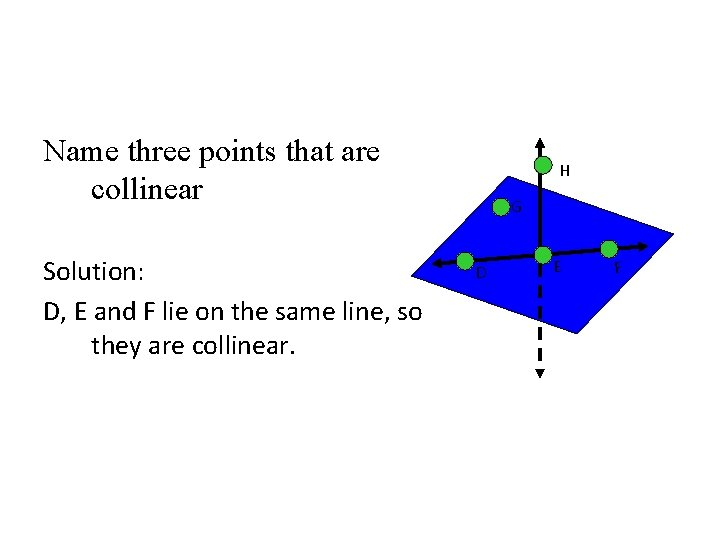Name three points that are collinear Solution: D, E and F lie on the same line, so they are collinear. H G D E F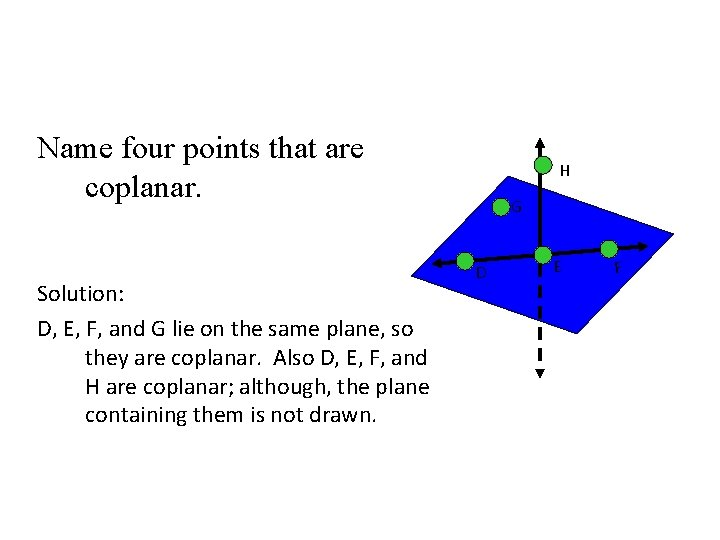Name four points that are coplanar. Solution: D, E, F, and G lie on the same plane, so they are coplanar. Also D, E, F, and H are coplanar; although, the plane containing them is not drawn. H G D E F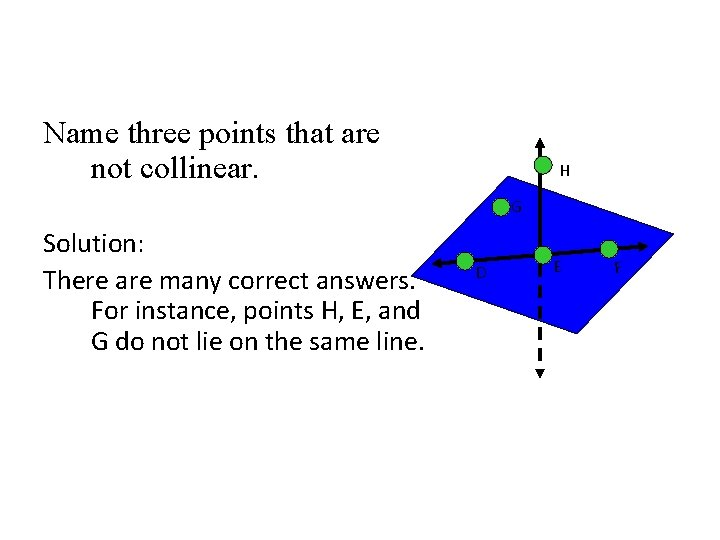Name three points that are not collinear. H G Solution: There are many correct answers. For instance, points H, E, and G do not lie on the same line. D E F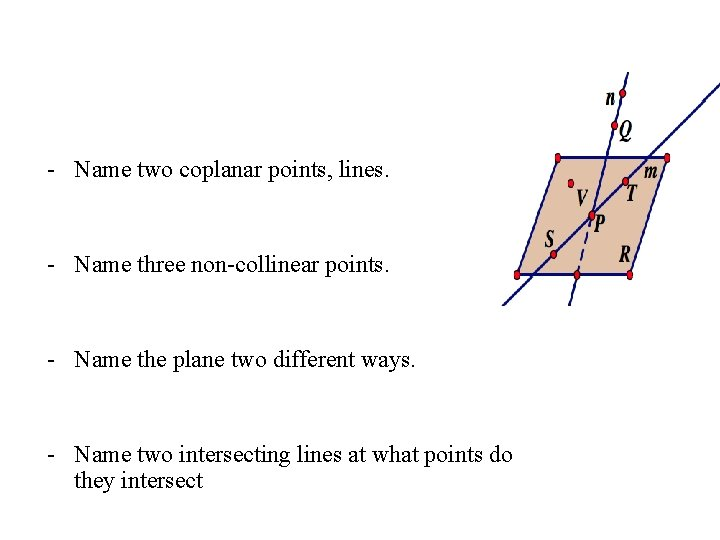- Name two coplanar points, lines. - Name three non-collinear points. - Name the plane two different ways. - Name two intersecting lines at what points do they intersect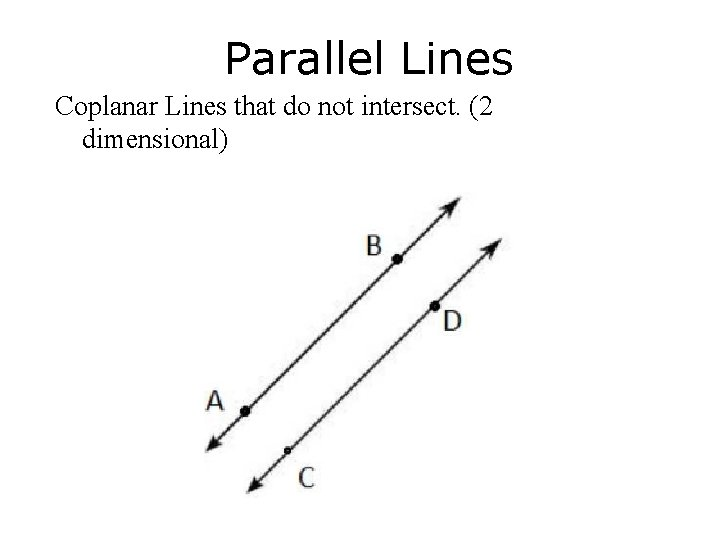Parallel Lines Coplanar Lines that do not intersect. (2 dimensional)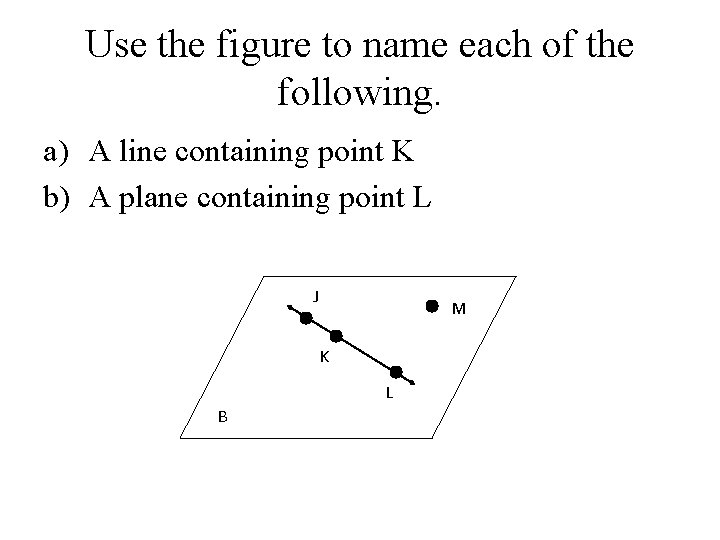Use the figure to name each of the following. a) A line containing point K b) A plane containing point L J M K L B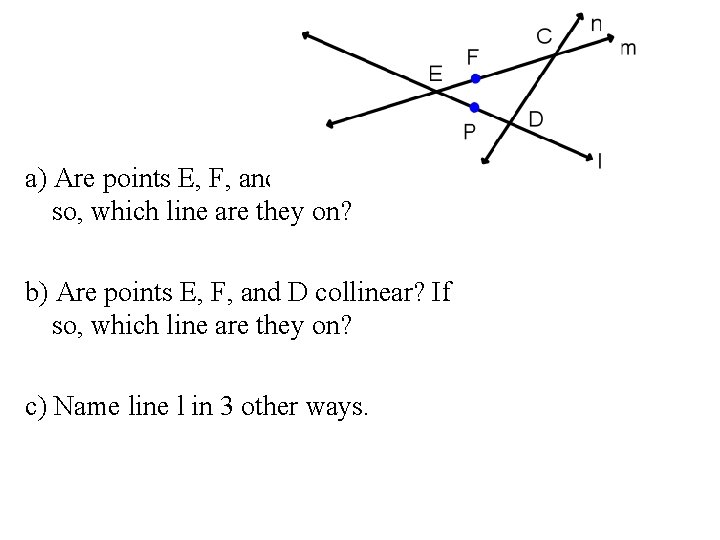Example 1 a) Are points E, F, and C collinear? If so, which line are they on? b) Are points E, F, and D collinear? If so, which line are they on? c) Name line l in 3 other ways.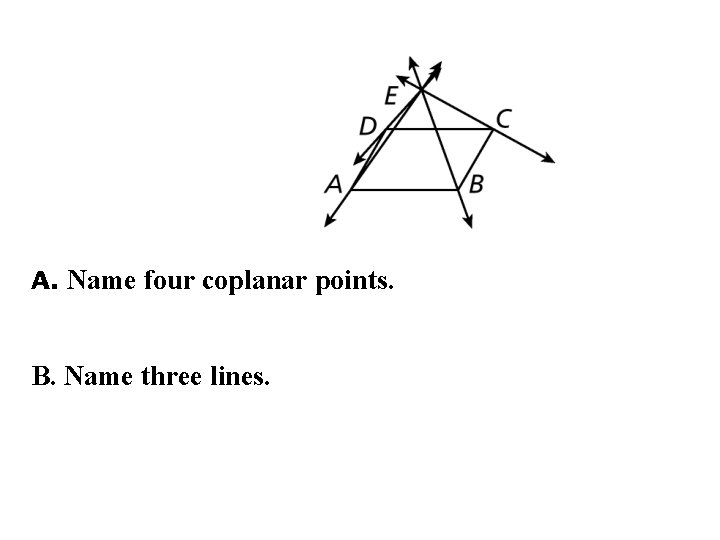le 2: A. Name four coplanar points. B. Name three lines.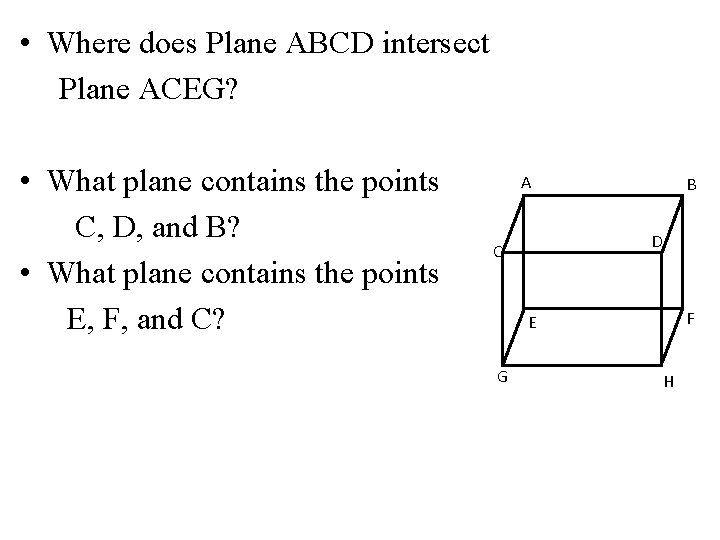• Where does Plane ABCD intersect Plane ACEG? • What plane contains the points C, D, and B? • What plane contains the points E, F, and C? A B D C F E G H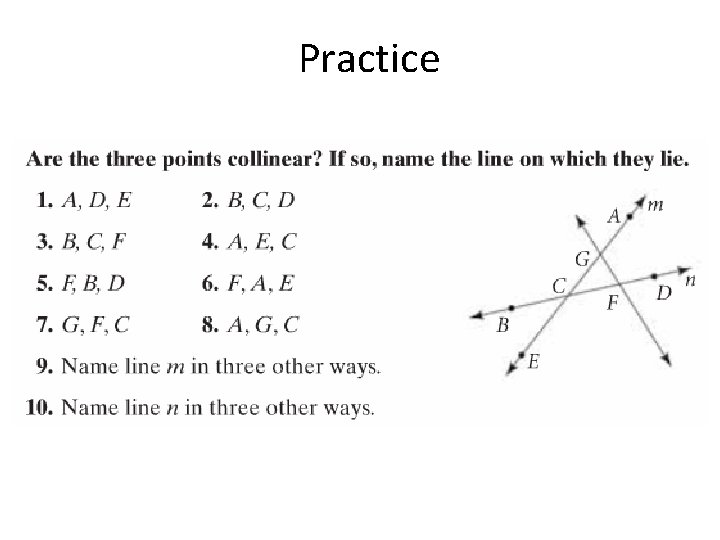PracticeExample 8: a) How many planes appear in this figure? A C B b) Name 3 points that are collinear. c) Are points A, B, C, and D coplanar? Explain. S D d) At what point does DB and CA intersect?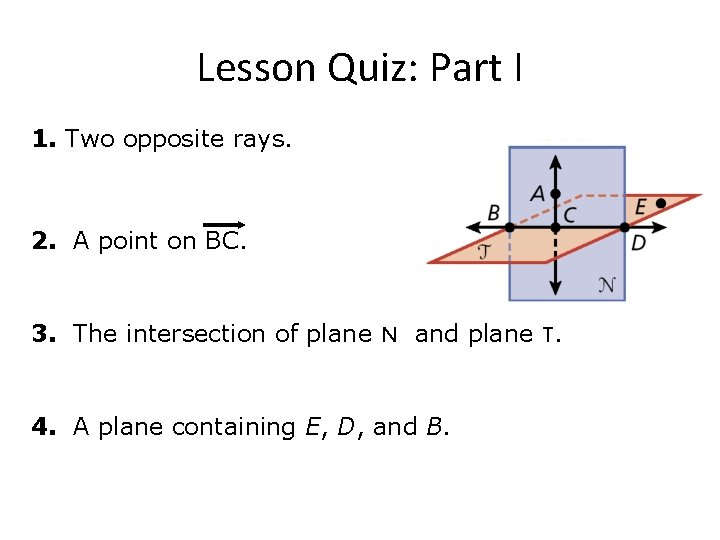Lesson Quiz: Part I 1. Two opposite rays. 2. A point on BC. 3. The intersection of plane N and plane T. 4. A plane containing E, D, and B.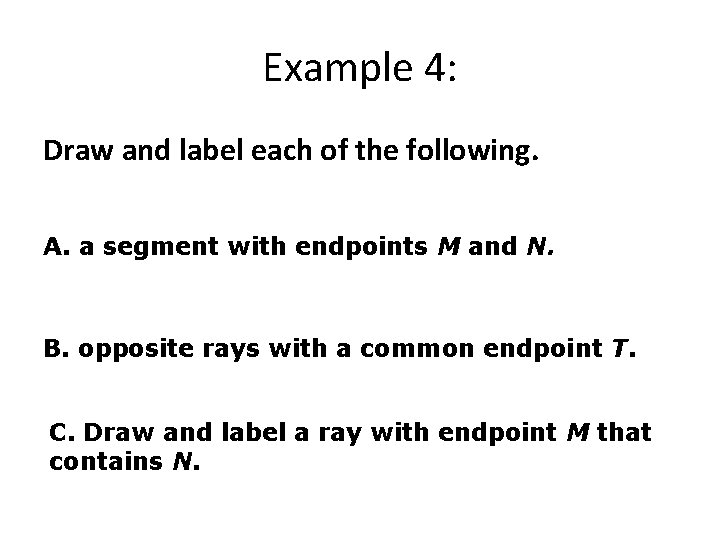Example 4: Draw and label each of the following. A. a segment with endpoints M and N. B. opposite rays with a common endpoint T. C. Draw and label a ray with endpoint M that contains N.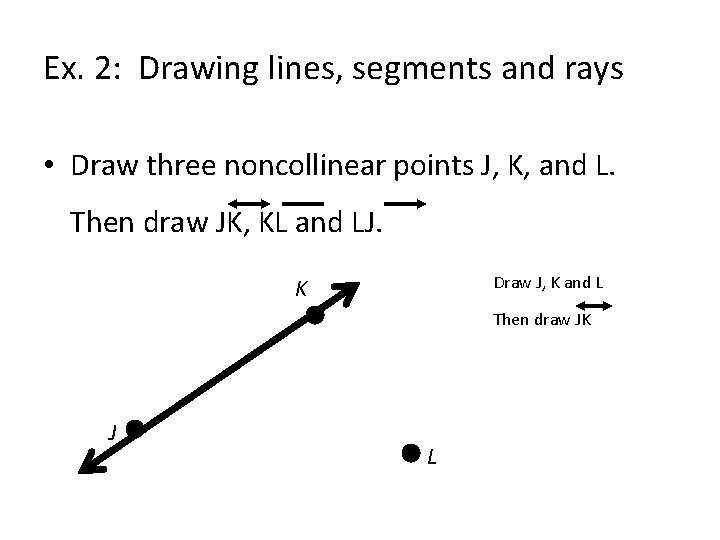Ex. 2: Drawing lines, segments and rays • Draw three noncollinear points J, K, and L. Then draw JK, KL and LJ. Draw J, K and L K Then draw JK J L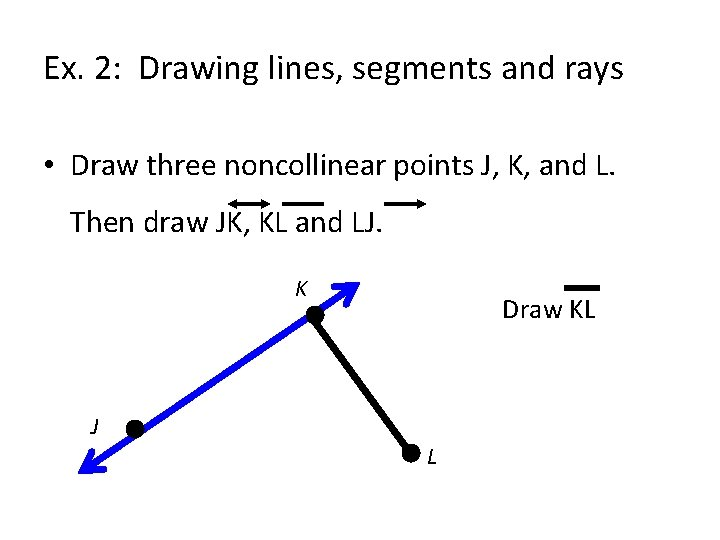Ex. 2: Drawing lines, segments and rays • Draw three noncollinear points J, K, and L. Then draw JK, KL and LJ. K Draw KL J LEx. 2: Drawing lines, segments and rays • Draw three noncollinear points J, K, and L. Then draw JK, KL and LJ. K Draw LJ J LEx. 3: Drawing Opposite Rays • Draw two lines. Label points on the lines and name two pairs of opposite rays. M Solution: Points M, N, and X Q X are collinear and X is between M and N. So XM and XN are opposite rays. P N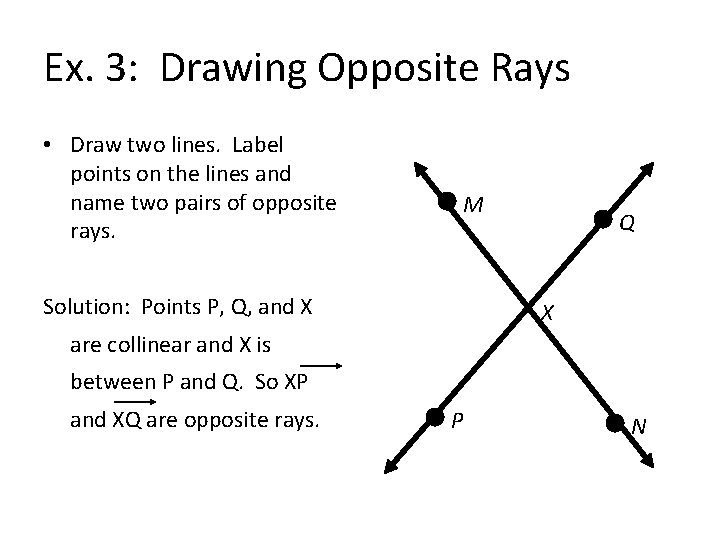Ex. 3: Drawing Opposite Rays • Draw two lines. Label points on the lines and name two pairs of opposite rays. M Solution: Points P, Q, and X Q X are collinear and X is between P and Q. So XP and XQ are opposite rays. P N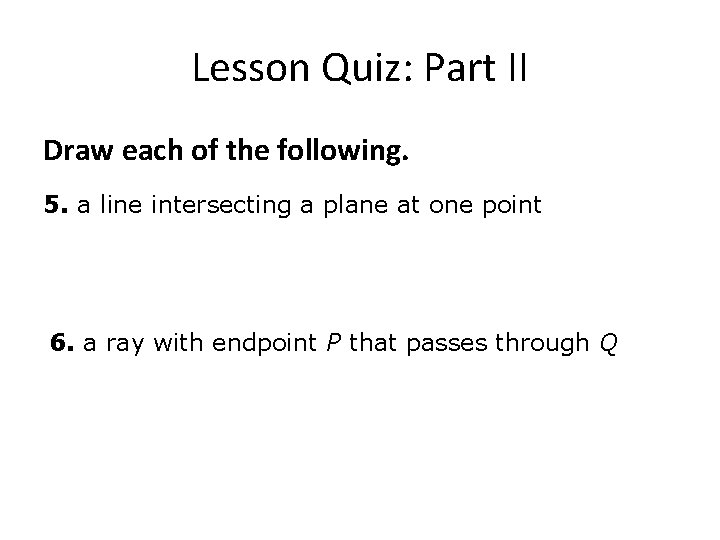Lesson Quiz: Part II Draw each of the following. 5. a line intersecting a plane at one point 6. a ray with endpoint P that passes through QEx. 4: Sketching intersections • a. Ø Ø Ø Sketch the figure described. A line that intersects a plane in one point Draw a plane and a line. Emphasize the point where they meet. Dashes indicate where the line is hidden by the plane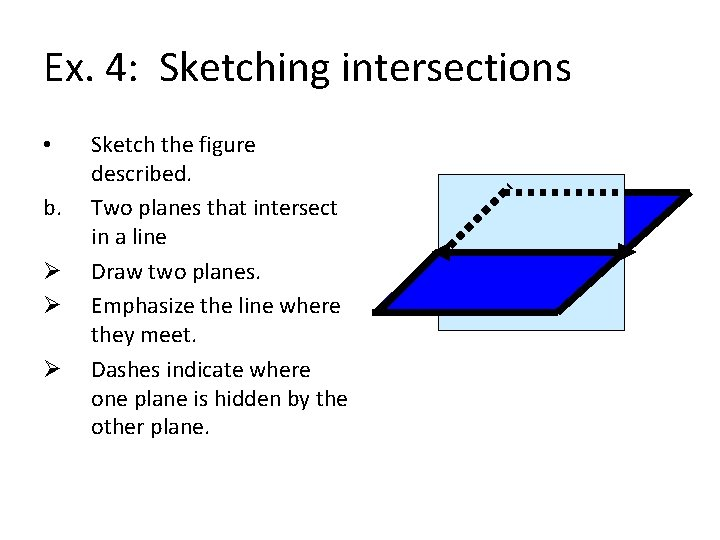Ex. 4: Sketching intersections • b. Ø Ø Ø Sketch the figure described. Two planes that intersect in a line Draw two planes. Emphasize the line where they meet. Dashes indicate where one plane is hidden by the other plane.